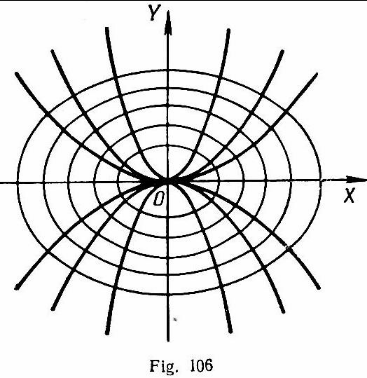# Orthogonal trajectory for  will be ( is parameter) Option 1) Option 2) Option 3) Option 4)

H Himanshu

As we have learnt,

Orthogonal Trajectory -

Any curve which cuts every member of a given family of curves at right angles

- whereinFirst of all we need differential equation for given family of curves. So, on differentiating we get -

Now if we replace  with , the resulting equation will be differential equation, for required orthogonal trajectory.

So we get -

Now, Integrating we get -

Option 1)

Option 2)

Option 3)

Option 4)

Exams
Articles
Questions